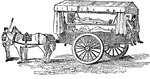Ambulance Cart

A cart used in the 19th century as an ambulance.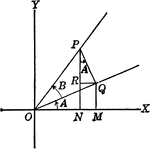Angles Used to Illustrate Sum and Difference of Two Angles

Angles used to illustrate the sum and difference of two angles and trig identities.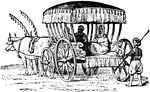Araba

"A heavy, springless wagon, usually covered with a screen as shelter from the rays of the sun, drawn…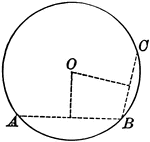Circle With Circumference Through Three Points

Illustration of a circle which illustrates that through three points not in a straight line one circumference,…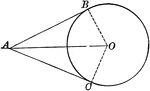Circle With Two Tangents Drawn From an External Point

Illustration of a circle which illustrates that the tangents to a circle drawn from an external point…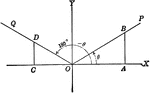Coordinate Axis With Angles, Lines, and Perpendiculars Drawn

Coordinate axis with angle XOP equal to theta, Θ, and angle XOQ=180 - Θ. From any point in the terminal…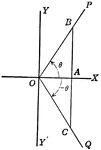Coordinate Axis With Perpendiculars Drawn To Form Similar Right Triangles From Positive and Negative Theta, Θ

Angle XOP=Θ and angle XOQ=- Θ. From a point in the terminal side of each a perpendicular line is drawn…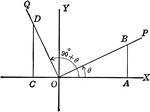Coordinate Axis With Perpendiculars Drawn To Form Similar Right Triangles

Angle XOP=Θ and angle XOQ=90+Θ. From a point in the terminal side of each a perpendicular line is…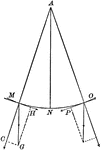Motion of a Pendulum

"When the pendulum is drawn from its vertical position, the force of gravity, MG, is resolved into two…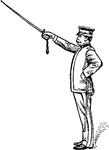Drawn Saber

"When publishing orderes, calling the roll, etc., the saber is held suspended from the right wrist by…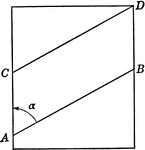Spiral Drawn on Piece of Paper Shown Flat

Spiral with lines AB and CD drawn on piece of paper shown flat.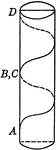Spiral Drawn on Piece of Paper and Rolled

Spiral with lines AB and CD drawn on piece of paper and rolled into a cylinder to form spiral running…Triangle ABC With Height h

Triangle ABC with sides a,b,c labeled and angles A,B,C labeled and h labeled as height.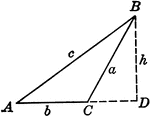Obtuse Triangle ABC With Height h

Triangle ABC with sides a,b,c labeled and angles A,B,C labeled and h labeled as height.Right Triangle OCB With, x, y, and r shown

Right triangle OCB that can be used to show the relationships between x, y, r, and Θ.